# Topic 5 Flashcards Preview

## IB SL Physics > Topic 5 > Flashcards

Flashcards in Topic 5 Deck (73):
1

2

3

4

5

6

7

8

9

10

11

12

13

14

15

16

17

18

19

20

21

22

23

24

25

26

27

28
29

30

31

32

33

34

35

## In the diagram the voltmeter V has a resistance of 100 kΩ and is connected such as to measure the potential difference across the 100 kΩ resistor. The battery has an emf of 5.0 V and negligible internal resistance.The reading on the voltmeter will beA. 4.0 V.B. 2.5 V.C. 1.0 V.D. zero.36

37

38

39

## In a laboratory experiment, a variable voltage power supply is used to provide voltage versus current data for two unequal resistors. These two resistors are first connected separately to the power supply, then as a series combination and then as a parallel combination. The four sets of results are plotted as shown.Which set of results would correspond to the two resistors connected in parallel?A. 1B. 2C. 3D. 440

41

## Two resistors in parallel form R1 and R2 part of a circuit. has R1 three times the resistance of , and R2 the total current flowing into the parallel pair is 12 A as shown.42

## A simple form of lighting for decorating trees sometimes consists of a string of small light bulbs connected in series as shown.With this simple system, if one of the bulbs burns out (its filament breaks) then the others willA. all go out.B. glow dimmer than before.C. glow just as bright as before.D. glow brighter than before.43

44

## A student constructs a ìvoltage dividerî which will provide an output voltage of 6 V from an input voltage of 9 V. She connects two resistors and as shown.The ratio of the resistances R1: R2 must be A. 1 : 2 .B. 2 : 1 .C. 2 : 3 .D. 3 : 2 .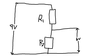45

## The graph below shows the current/voltage characteristics of a filament lamp.The resistance of the filament at 4.0 V isA. 250 ohms.B. 4000 ohms.C. 8000 ohms.D. 64000 ohms.46

47

48

## A cell of e.m.f. E and internal resistance r is connected to a variable resistor. A voltmeter is connected so as to measure the potential difference across the terminals of the cell. Which one of the following is the correct circuit diagram of the arrangement?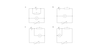49

50

## Which one of the following shows a correct circuit, using ideal voltmeters and ammeters, for measuring the I-V characteristic of a filament lamp?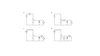51

52

53

## In the circuit below, which meter is not correctly connected?A. 1B. 2C. 3D. 454

55

## In the circuit below, n charge carriers pass the point P in a time t. Each charge carrier has charge q.A. q/tB. nq/tC. qt/nD. nqt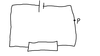56

57

58

## The diagrams below show combinations X, Y and Z of three resistors, each resistor having the same resistance. Which one of the following shows the resistances of the combinations in increasing order of magnitude?59

## A battery of e.m.f. E and negligible internal resistance is connected to three resistors, each of resistance R, a voltmeter and a switch, as shown below. The voltmeter has infinite resistance.What are the readings on the voltmeter when the switch is open and when it is closed?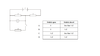60

## The graph below shows the variation with voltage V of the current I in three resistors X, Y and ZWhich of the following corresponds to resistors for which the resistance increases with increasing current?A. X onlyB. Z onlyC. X and ZD. Y and Z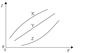61

62

## In the two circuits X and Y below, each cell has an e.m.f. E and negligible internal resistance. Each resistor has a resistance R.The power dissipated in circuit X is P. The best estimate for the power dissipated in circuit Y isA. P/4 .B. P/2 .C. 2P.D. 4P.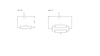63

64

## A battery is connected to a resistor as shown belowThe battery transfers energy EB when charge Q passes completely around the circuit and the resistor transfers energy ER. The e.m.f. of the battery is equal toA. ER/QB. EB/QC. (EB + ER)/QD. (EB + ER)/Q65

## In the circuit shown below, the cell has negligible internal resistance.Which of the following equations is correct?A. I1=2I2B. I1=2I3C. I2=2I3D. I3=2I166

## The graph below shows the variation with potential difference V of the current I in an electrical component.Which one of the following is a correct statement about the resistance of the component?A. For potential differences greater than V0, the resistance is constant.B. For potential differences greater than V0, the resistance decreases with increasing potential difference.C. The variation of current with potential difference is linear and so Ohm’s law is obeyed.D. For potential differences less than V0, the resistance is zero.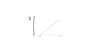67

## In which one of the circuits is it possible to vary the current in the lamp by adjusting the variable resistor? The cell has negligible internal resistance.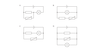68

69

70

## A resistor of resistance 1.0 ohms is connected in series with a battery. The current in the circuit is 2.0 A. The resistor is now replaced by a resistor of resistance of 4.0 ohms. The current in this circuit is 1.0 A.The best estimate for the internal resistance of the battery isA. 1.0 ohms.B. 2.0 ohms.C. 4.0 ohms.D. 5.0 ohms.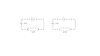71

72

## The graphs below are the current-voltage (I-V) characteristics of three electrical components P, Q and R.Which component(s) has (have) constant resistance?A. P onlyB. R onlyC. P and Q onlyD. P and R only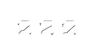73

## In the circuit below, resistors X, Y and Z are connected in series with a 9.0 V supply.Resistors X and Z are fixed resistors of resistance 3000 ohms. The resistance of resistor Y may be varied between zero and 3000 ohms. Which of the following gives the maximum range of potential difference V across the resistors X and Y?A. 0 to 6.0VB. 3.0V to 6.0VC. 4.5V to 6.0VD. 4.5V to 9.0V